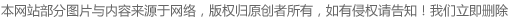010-68815806

330809108Research Program Summary

At August  I had the honor to participate in a research based program. In this program, I had an opportunity to learn about data analysis and machine learning. In the following summary will be the detailed report of this program. What I can said is that, I learn many things from this program and this will be an extremely valuable experience in my life.STEP ONE
At the start of the first week, we have a brief introduction to our fellow program participant: a senior (high school) from Los Angeles, a sophomore (high school) from Minnesota, and the mentor of our program-Dr.C, PHD of UC Berkeley. In the first meeting, Dr.Chang elaborate the basic definition of machine learning and the project we are going to do. The project we about to challenge is a kaggle competition, which we need to create a model that can predict the real estate price’s error. At the first glance, the project seems like mission impossible, but Dr.C has already have a plan to help us to accomplish this goal.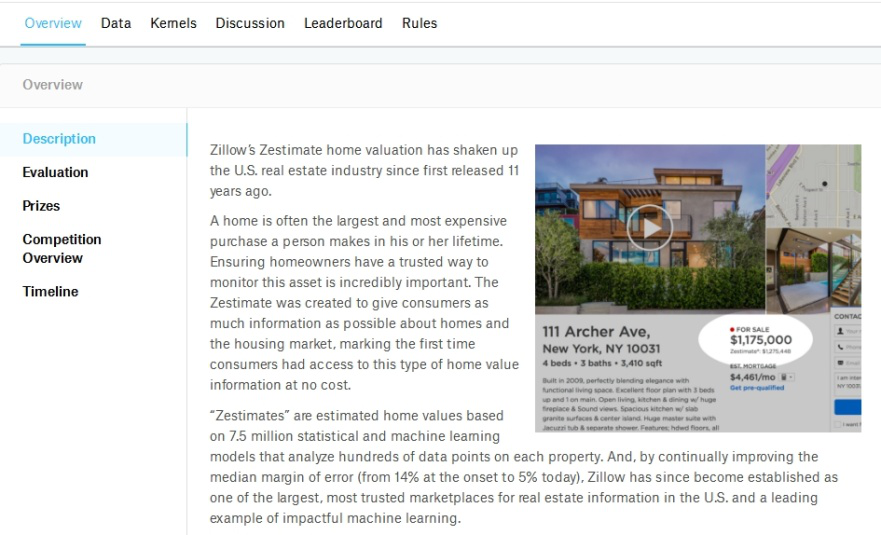Here is the link on our project and schedule:

Lecture for the introduction of ML:

The assignment we need to do is to read machine learning basics (chapter 5), and python environment setup. (the computer language we use for our project is python)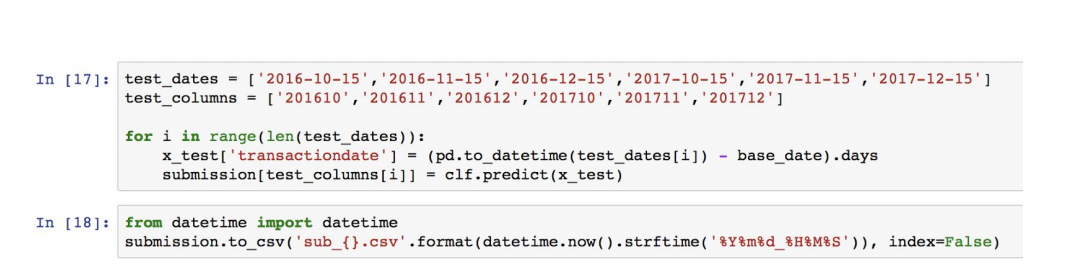After the setup, Dr.C walks us through the procedures of making the prediction model. First we need to import the necessary modules. The modules include: numpy, scikit learn, matplotlib, panda and csv files, which allow us to use functions that are pre-build in these modules.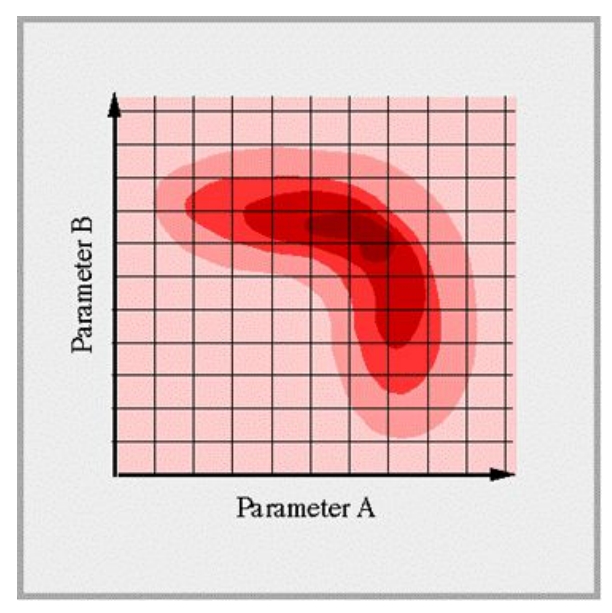The first thing we need to do is to import the data. While the csv is imported, we can proceed to clean the data. The significance of the data cleaning is to make sure the accurateness of our data. As we load the data into our model, we want the model to give valid prediction, which required a good training set for the computer to learn the function.

The process of data cleaning was not easy for us. The reasons for this is we do not have enough background knowledge nor experience with python and machine learning. With help of Dr.C, we managed to overcome the obstacles and finish the data cleaning.
Here is the part of data provided by the zillow price:

Modules that we used:

http://www.numpy.org/
http://pandas.pydata.org/

STEP Two
After the data cleaning process, we need to import the necessary model or algorithm to help the computer create a predictive function, the first function we apply is a linear regression module from scikit learn. What the linear regression do is from all the training set it will draw a linear function to fits the x inputs and y outputs.
Here is the what the code looks like:

from sklearn.linear_model import LinearRegression

After the computer gave the results, we put the results into the csv format and submit to the competition:

Well, the ranking for my submission is not that great using the linear regression, it place me around 1250th, so that is when the boosted trees comes in.
Noted that many of this algorithms and functions are based on mathematical principles, as Dr.C taught us the math behind machine learning.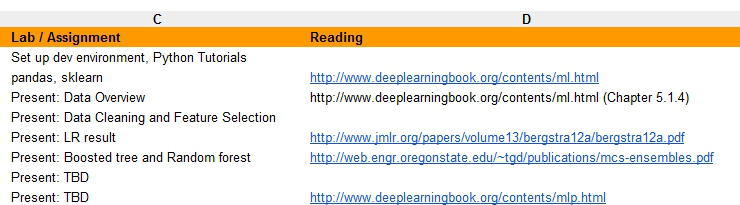Boosted trees is a machine technique for regression and classification problems, which produces a prediction model in the form of an ensemble of weak prediction models, typically decision. It builds the model in a stage-wise fashion like other boosting methods do, and it generalizes them by allowing optimization of an arbitrary differentiable cost function.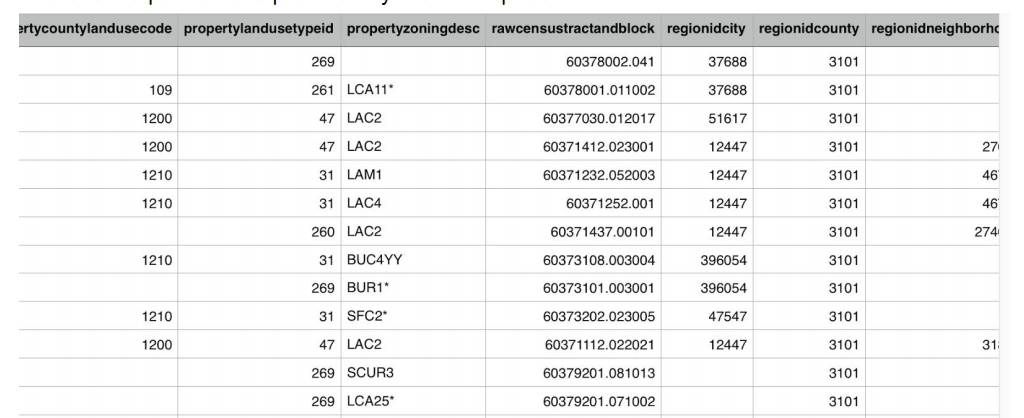The boosted tree algorithm that we first adapted is xgboost, short for “Extreme Gradient Boosting”. We believe that if we use xgboost instead of linear regression, we can get a better model.

To use xgboost, there is couple hyperparameters that we need to tune: learning rate (steps that the computer take when performing gradient descent), max depth (control/prevent the overfitting), and subsample (also control overfitting or underfitting, fraction of observation that are randomly samples.) In order to tune for the best hyperparameter, another concept need to be apply: grid search and random forest search.

Grid search and random forest search can be put this simply, the specific type of algorithm that help the us identify the best hyperparameters to use.
Here is what a grid search looks like:?

By using the grid search, we able to find the best parameter, as I apply the xgboost as my module, my ranking went from 1200 ish to 700 ish. We can see the significance change due the change of model.

Currently the model I’m using is 丨ightBGM, recommend by Dr.C , the model is more efficient and accurate than xgboost. The process is not easy to us beginners, but I believe that there is a lot of things we can learn from this process, and can be handy to us in the futures.